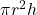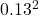## Your office has a 0.025 m^3 cylindrical container of drinking water. The radius of the container is about 13 cm. Required:a. When the contai

Question

Your office has a 0.025 m^3 cylindrical container of drinking water. The radius of the container is about 13 cm. Required:a. When the container is full, what is the pressure that the water exerts on the sides of the container at the bottom of the container above the atmospheric pressure? b. When the container is full, what is the pressure that the water exerts on the sides of the container halfway down from the top above the atmospheric pressure?

in progress 0
7 months 2021-07-24T19:23:23+00:00 1 Answers 3 views 0

## Answers ( )

a) 105935.7 Pa

b) 103630.35 Pa

Explanation:

The volume of the container = 0.025 m^3

The radius of the container = 13 cm = 0.13 m

We have to find the height of the tank

From the equation for finding the volume of the cylinder,

V =where

V is the volume of the cylinder

h is the height of the cylinder

substituting values, we have

0.025 = 3.142 xx h

0.025 = 0.0531h

h = 0.025/0.0531 = 0.47 m

Pressure at the bottom of the tank P = ρgh

where

ρ is the density of water = 1000 kg/m^3

g is the acceleration due to gravity = 9.81 m/s^2

h is the depth of water which is equal to the height of the tank

substituting values, we have

P = 1000 x 9.81 x 0.47 = 4610.7 Pa

atmospheric pressure = 101325 Pa

therefore, the pressure in the tank bottom above atmospheric pressure = 101325 Pa + 4610.7 Pa = 105935.7 Pa

b) For half way down the container, depth of water will be = 0.47/2 = 0.235 m

pressure P = 1000 x 9.81 x 0.235 = 2305.35 Pa

This pressure above atmospheric pressure = 101325 Pa + 2305.35 Pa = 103630.35 Pa